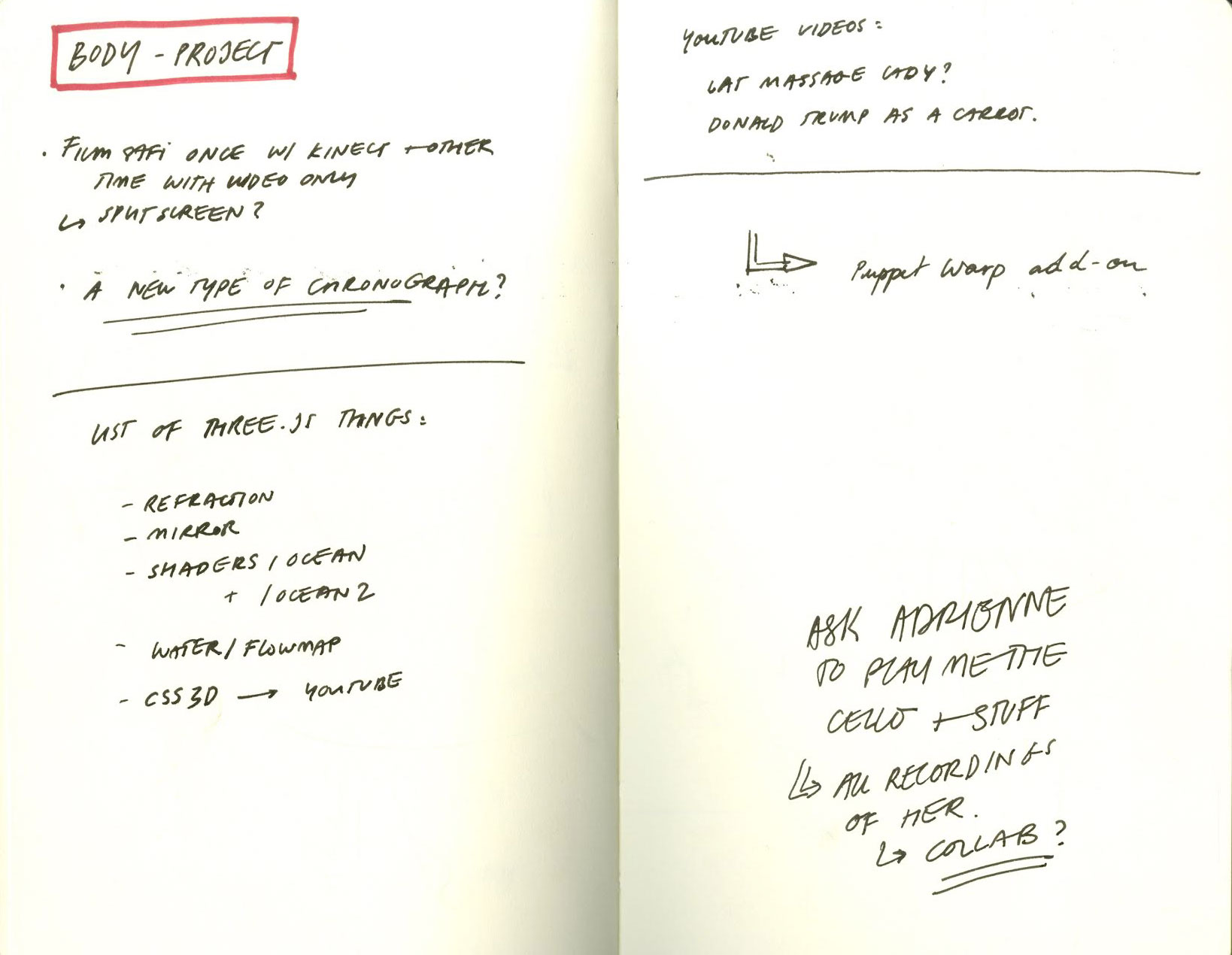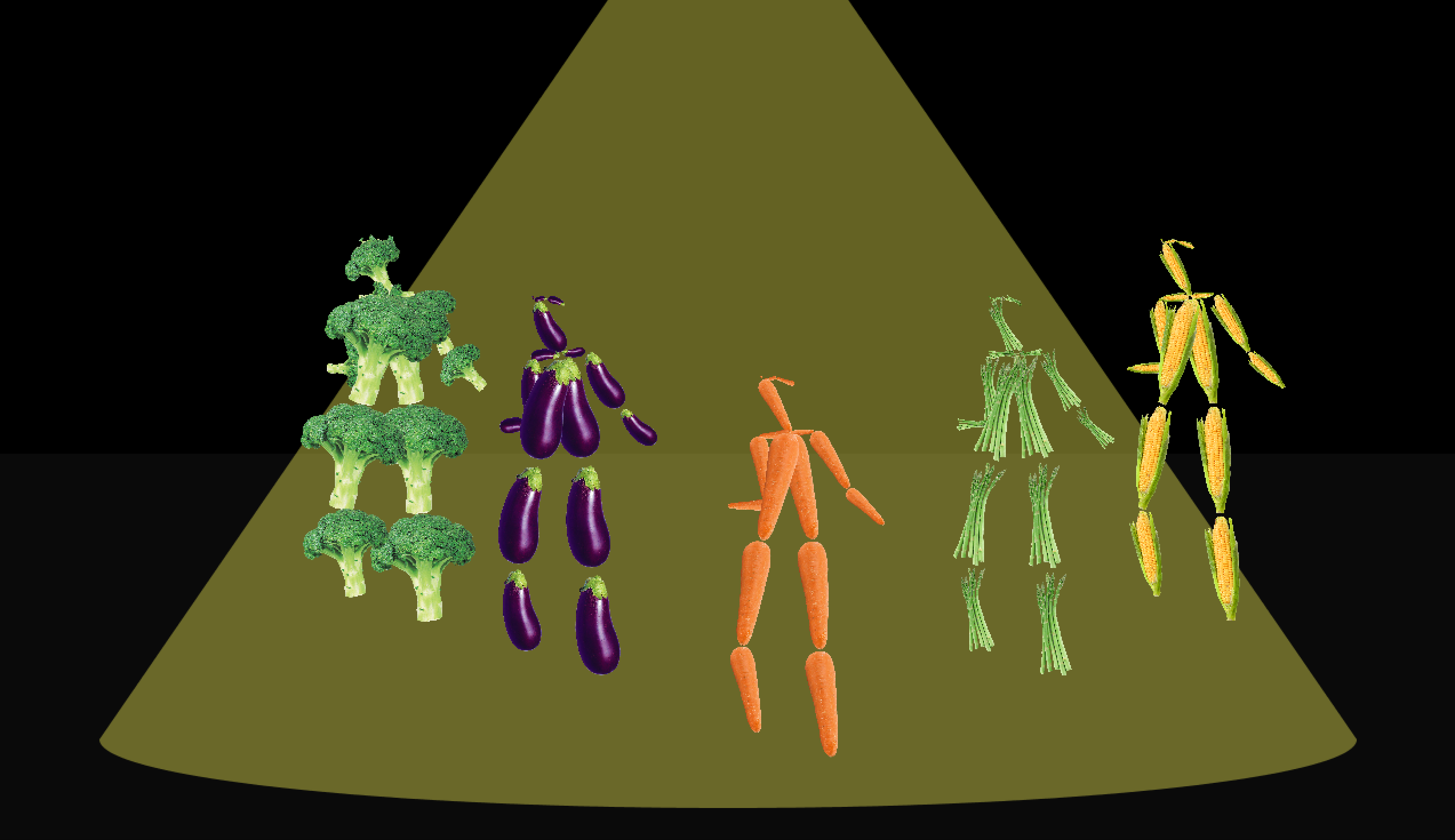# dechoes – Mocap

Veggie PartyFor this project, I really wanted to use OpenPose, which is a brand new, off the shelf piece of software developed by CMU School of Computer Science. Unlike RGBD recordings, OpenPose is able to extract skeletons from already existing 2D videos and saves all of the body coordinates to a JSON file.

I started this project with some very large scale ideas, which I quickly realized were not achievable in a couple days. I finally settled on the idea of a Veggie Party, where each character was made of a different vegetable, mapped to its limbs.

In the final outcome, there was no specific reason to use OpenPose since the movement is quite mundane. I had originally wanted to use an already existing video of people pealing or cutting vegetables (which would have generated a never ending cycle of veggies, pealing and eating other veggies), but that specific source material could not be found. Additionally, the OpenPose data is still extremely flawed, but I decided to just roll with it and embrace the experimental glitch.

I cannot say that I am specifically psyched about this outcome, however I am excited to have been exposed to MoCap technology and am already thinking about larger scale projects I will be able to produce in the next few weeks.

Video:

Preliminary Notes:Background tries:Code:

```PImage carrot; PImage asparagus; PImage corn; PImage kale; PImage aubergine; PImage broccoli; PImage meat;   class SceneSequence { int sceneIndex = 0; int numScenes; int imageWidth, imageHeight; String poseModel; JSONObject[][] poses; public SceneSequence(JSONArray baseJSON) { JSONObject baseObj = baseJSON.getJSONObject(0); numScenes = baseObj.getInt("numScenes"); poses = new JSONObject[numScenes][]; imageWidth = baseObj.getInt("imageWidth"); imageHeight = baseObj.getInt("imageHeight"); poseModel = baseObj.getString("poseModel"); for (int sceneIndex = 0; sceneIndex &lt; numScenes; sceneIndex++) { JSONObject scene = baseObj.getJSONObject("" + sceneIndex); int numPoses = scene.getInt("numPoses"); poses[sceneIndex] = new JSONObject[numPoses]; for (int poseIndex = 0; poseIndex &lt; numPoses; poseIndex++) { JSONObject pose = scene.getJSONObject("" + poseIndex); poses[sceneIndex][poseIndex] = pose; } } } public void drawFrame() {   JSONObject[] scene = poses[sceneIndex]; for (int i = 0; i &lt; scene.length; i++) { JSONObject pose = scene[i]; println(poseModel); if (poseModel.equals("COCO")) drawCOCOPose(pose); else drawMPIPose(pose); } sceneIndex++; if (sceneIndex &gt;= numScenes) sceneIndex = 0; } }   void connectJoints(JSONObject pose, String joint0, String joint1) {   JSONArray pt0 = pose.getJSONArray(joint0); JSONArray pt1 = pose.getJSONArray(joint1); float x0 = pt0.getFloat(0); float y0 = pt0.getFloat(1); float x1 = pt1.getFloat(0); float y1 = pt1.getFloat(1); float x2 = x0 + 200; float y2 = y0 - 70; float x3 = x1 + 200; float y3 = y1 - 70; float x4 = x0 - 200; float y4 = y0 - 70; float x5 = x1 - 200; float y5 = y1 - 70; float x6 = x0 - 350; float y6 = y0 - 120; float x7 = x1 - 350; float y7 = y1 - 120; float x8 = x0 + 350; float y8 = y0 - 120; float x9 = x1 + 350; float y9 = y1 - 120;   if ((x0 != 0) &amp;&amp; (y0 != 0) &amp;&amp; (x1 != 0) &amp;&amp; (y1 != 0)) { if ((x0 != 0) &amp;&amp; (y0 != 0) || (x1 != 0) &amp;&amp; (y1 != 0)) { // line(x0, y0, x1, y1);   drawCarrotOnLimb(x0, y0, x1, y1); drawAsparagusOnLimb(x2, y2, x3, y3); drawAubergineOnLimb(x4, y4, x5, y5); drawBroccoliOnLimb(x6, y6, x7, y7); drawCornOnLimb(x8, y8, x9, y9); } } }     void drawMPIPose(JSONObject pose) {   connectJoints(pose, "head", "neck"); connectJoints(pose, "neck", "left_shoulder"); connectJoints(pose, "neck", "right_shoulder"); connectJoints(pose, "neck", "left_hip"); connectJoints(pose, "neck", "right_hip"); connectJoints(pose, "left_shoulder", "left_elbow"); connectJoints(pose, "left_elbow", "left_hand"); connectJoints(pose, "right_shoulder", "right_elbow"); connectJoints(pose, "right_elbow", "right_hand"); connectJoints(pose, "left_hip", "left_knee"); connectJoints(pose, "left_knee", "left_foot"); connectJoints(pose, "right_hip", "right_knee"); connectJoints(pose, "right_knee", "right_foot"); }   void drawCOCOPose(JSONObject pose) { connectJoints(pose, "left_eye", "nose"); connectJoints(pose, "right_eye", "nose"); connectJoints(pose, "left_ear", "left_eye"); connectJoints(pose, "right_ear", "right_eye"); connectJoints(pose, "nose", "neck"); connectJoints(pose, "neck", "left_shoulder"); connectJoints(pose, "left_shoulder", "left_elbow"); connectJoints(pose, "left_elbow", "left_wrist"); connectJoints(pose, "neck", "right_shoulder"); connectJoints(pose, "right_shoulder", "right_elbow"); connectJoints(pose, "right_elbow", "right_wrist"); connectJoints(pose, "neck", "left_hip"); connectJoints(pose, "left_hip", "left_knee"); connectJoints(pose, "left_knee", "left_foot"); connectJoints(pose, "neck", "right_hip"); connectJoints(pose, "right_hip", "right_knee"); connectJoints(pose, "right_knee", "right_foot"); }     JSONArray baseJSON; SceneSequence sequence;   void setup() { size(1280, 740); stroke(255); baseJSON = loadJSONArray("dance2.json"); sequence = new SceneSequence(baseJSON); carrot = loadImage("data/Carrot.png"); asparagus = loadImage("data/Asparagus.png"); corn = loadImage("data/Corn.png"); kale = loadImage("data/Kale.png"); aubergine = loadImage("data/Aubergine.png"); broccoli = loadImage("data/Broccoli.png"); meat = loadImage("data/meat.jpg"); }   void draw() { background(0); //drawBackground(); //spotlight(); sequence.drawFrame(); fill(255); //text(frameCount, mouseX, mouseY); }     void drawCarrotOnLimb(float x0, float y0, float x1, float y1) {   float cw = carrot.width; float ch = carrot.height; float carrotLength = ch; float desiredLength = dist(x0, y0, x1, y1); float ratio = desiredLength / carrotLength;   float dy = y1 - y0; float dx = x1 - x0; float orientation = atan2(dy, dx);   pushMatrix(); translate(x0, y0); scale(ratio, ratio); rotate(orientation - HALF_PI); translate(0-cw/2, 0); image(carrot, 0, 0); popMatrix(); }     void drawAsparagusOnLimb(float x2, float y2, float x3, float y3) { float cw = asparagus.width; float ch = asparagus.height; float asparagusLength = ch; float desiredLength = dist(x2, y2, x3, y3); float ratio = desiredLength / asparagusLength;   float dy3 = y3 - y2; float dx3 = x3 - x2; float orientation = atan2(dy3, dx3);   pushMatrix(); translate(x2, y2); scale(ratio, ratio); rotate(orientation - HALF_PI); translate(0-cw/2, 0); image(asparagus, 0, 0); popMatrix(); }     void drawAubergineOnLimb(float x4, float y4, float x5, float y5) { float cw = aubergine.width; float ch = aubergine.height; float aubergineLength = ch; float desiredLength = dist(x4, y4, x5, y5); float ratio = desiredLength / aubergineLength;   float dy5 = y5 - y4; float dx5 = x5 - x4; float orientation = atan2(dy5, dx5);   pushMatrix(); translate(x4, y4); scale(ratio, ratio); rotate(orientation - HALF_PI); translate(0-cw/2, 0); image(aubergine, 0, 0); popMatrix(); }   void drawBroccoliOnLimb(float x6, float y6, float x7, float y7) { float cw = broccoli.width; float ch = broccoli.height; float broccoliLength = ch; float desiredLength = dist(x6, y6, x7, y7); float ratio = desiredLength / broccoliLength;   float dy7 = y7 - y6; float dx7 = x7 - x6; float orientation = atan2(dy7, dx7);   pushMatrix(); translate(x6, y6); scale(ratio, ratio); rotate(orientation - HALF_PI); translate(0-cw/2, 0); image(broccoli, 0, 0); popMatrix(); }   void drawCornOnLimb(float x8, float y8, float x9, float y9) { float cw = corn.width; float ch = corn.height; float cornLength = ch; float desiredLength = dist(x8, y8, x9, y9); float ratio = desiredLength / cornLength;   float dy9 = y9 - y8; float dx9 = x9 - x8; float orientation = atan2(dy9, dx9);   pushMatrix(); translate(x8, y8); scale(ratio, ratio); rotate(orientation - HALF_PI); translate(0-cw/2, 0); image(corn, 0, 0); popMatrix(); }   void drawBackground(){ image(meat, 0, 0); pushMatrix(); fill(0, 0, 0, 100); rect(0, 0, 1280, 740); popMatrix();   }   void spotlight(){ noStroke(); fill(10, 10, 10); rect(0, 400, width, 500); fill(255, 249, 89, 100); arc(width/2, 650, 1100, 120, 0, PI, OPEN); triangle(width/2, -150, width/2 - 550, 650, width/2 + 550, 650); }```